## The force of 20\hat{j}~\text{N}20 ​j ​^ ​​ N is applied at \vec{r} =(4.0\hat{i}-2.0\hat{j})~\text{m} ​r ​⃗ ​​ =(4.0 ​i ​^ ​​ −2.0 ​j ​^ ​​ )

Question

The force of 20\hat{j}~\text{N}20 ​j ​^ ​​ N is applied at \vec{r} =(4.0\hat{i}-2.0\hat{j})~\text{m} ​r ​⃗ ​​ =(4.0 ​i ​^ ​​ −2.0 ​j ​^ ​​ ) m. What is the torque of this force about the origin?

in progress 0
5 months 2021-09-01T03:10:49+00:00 1 Answers 0 views 0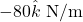Explanation:

Torque is given by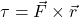Note that this is a cross product of vectors. To perform a cross product, we need to express the vectors in three components.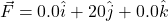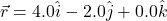The torque is then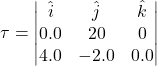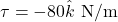(I’m not sure if the matrix will appear well because I’m constrained with the device I’m using but what I’m showing is the determinant of a matrix with i, j, k in the first row, the coefficients of F in the second and the coefficients of r in the third)